# Three Phase Induction Motors

## Three Phase Induction Motors:

Three Phase Induction Motors are of two types: squirrel-case and wound-rotor. In squirrel-cage, the rotor consists of longitudinal conductor-bars shorted by circular connectors at the two ends while in wound-rotor motor, the rotor also has a balanced three-phase distributed winding having same poles as stator winding. However, in both, stator carries a three-phase balanced distributed winding.

### Analysis and Performance:

Per-phase equivalent circuit of a Three Phase Induction Motors is shown in Fig. 6.1(a). R′r and X′r are the stator referred values of rotor resistance Rr and rotor reactance Xr. Slip is defined by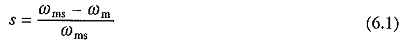where ωm and ωms are rotor and synchronous speeds, respectively. Furtherwhere f and p are supply frequency and number of poles, respectively.

Since, stator impedance drop is generally negligible compared to terminal voltage V, the equivalent circuit can be simplified to that shown in Fig. 6.1(b).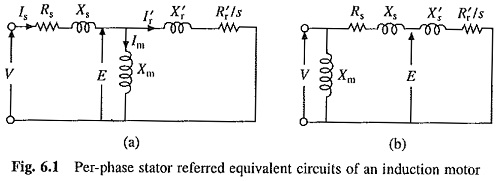Also from Eq. (6.1)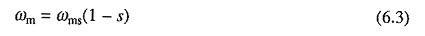From Fig. 6.1(b),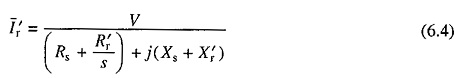Power transferred to rotor (or air-gap power)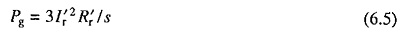Rotor copper loss is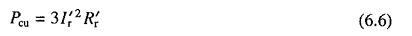Electrical power converted into mechanical powerTorque developed by motor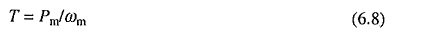Substituting from Eqs. (6.3) and (6.7) yieldsSubstituting from Eq. (6.4) gives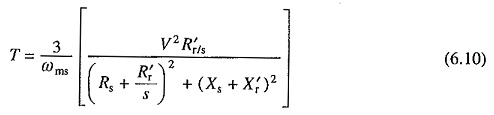A comparison of Eqs. (6.5) and (6.9) suggests that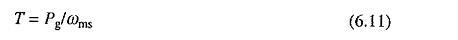Motor output torque at the shaft is obtained by deducting friction windage and core-loss torques from the developed torque.

The developed torque is a function of slip only (Eq. (6.10)). Differentiating T in (6.10) with respect to s and equating to zero gives the slip for maximum torque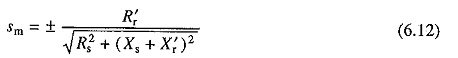Substituting from Eq. (6.12) into (6.10) yields an expression for maximum torque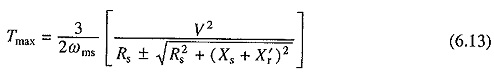Maximum torque is also known as breakdown torque. While it is independent of rotor resistance, Sm is directly proportional to rotor resistance.

The natures of speed-torque and speed-rotor current characteristics are shown in Fig. 6.2. Both rotor-current and torque are zero at synchronous speed. With decrease in speed, both increase. While torque reduces after reaching breakdown value, the rotor-current continues to increase, reaching a maximum value at zero speed. Drop in speed from no load to full load depends on the rotor resistance. When rotor resistance is low, the drop is quite small, and therefore, motor operates essentially at a constant speed. The breakdown torque is a measure of short-time torque overload capability of the motor.

Motor runs in the direction of the rotating field. Direction of rotating field, and therefore, motor speed can be reversed by reversing the phase sequence. Phase sequence can be reversed by interchanging any two terminals of the motor.

Sometime, torque is expressed in terms of sm and Tmax, which not only facilitates calculations, but also enables a quick appreciation of nature of speed-torque characteristics. Dividing Eq. (6.10) by (6.13) and then substituting from (6.12) yields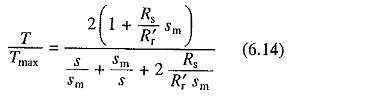The nature of speed-torque characteristics (Fig. 6.2) can now be readily explained from Eq. (6.14).For slips much smaller than sm, second term of the denominator dominates. Therefore, speed-torque relation from 0 to rated torque is approximately represented by a straight line. For slips much larger than sm, first term of the denominator dominates and speed-torque relation takes a hyperbolic shape in this region.

In the whole region of motor operation, term (Rssm/R′r) is small compared to 1 and dominating term in the denominator. Therefore, it can be dropped from Eq. (6.14). Thus### Induction Motors with Special Designs:

A general purpose induction motor is designed to operate at low slip at full load in order to have good running performance. Depending on the rating, full load slip varies from 2 to 7%. Such a motor has high starting current (5-8 times) and low starting torque (full load torque to twice Bill load torque). Certain applications require motor to be designed differently. Some of these are:

### High Slip Induction Motors:

For intermittent load applications, involving frequent start and stop and/or running at low speeds for prolonged periods, induction motors are designed with high rotor resistance. Such motors have low starting current and high starting torque, but low full load efficiency due to high rotor copper loss. Because these motors operate at a large slip (between 10 and 40% at full load) they are called high slip motors. High slip motors are also suitable for fan drives where speed is controlled by stator voltage control and are found among both—squirrel-cage and wound rotor. The nature of speed-torque characteristics of such motors is shown in Fig. 6.3(c).

In squirrel-case induction motors, good starting performance (low starting current and high starting torque) is realized without appreciably affecting full load performance by the use of deep-bar rotor or double-cage rotor motors.

Rotor frequency changes from 50 Hz to 1-3 Hz as the speed changes from standstill to full load: Variation of rotor frequency is utilized in these motors to vary rotor resistance from a large value at standstill to a very small value at full speed. Thus, while starting and low speed performance is improved, full load performance is not appreciably effected.

### Deep-Bar Squirrel-cage Rotor Induction Motor:

Stator of the machine is identical to a general purpose induction motor. Rotor has deep and narrow conductor bars as shown in Fig. 6.3(a).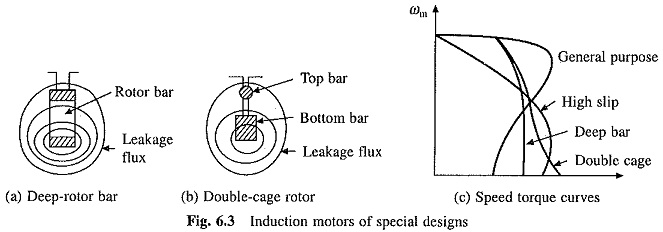Slot leakage fluxes produced by the current in bar are also shown in the figure. One can imagine that the bar is made of a number of narrow layers connected in parallel. Let us compare the behavior of top and bottom layers. More leakage flux links with bottom layer than the top layer. Consequently, bottom layer has a much higher leakage inductance than the top layer. As rotor frequency is high at low speeds, the reactance and impedance of bottom layer are much higher than the top layer. Therefore, at low speeds highest amount of current is carried by topmost layer and lowest by the bottom most. Because of unequal distribution of current across cross section of the bar, effective resistance of rotor is high and starting and low speed performance is improved. At near full load speed both—frequency of rotor current and leakage reactance are low. Therefore, current gets equally distributed across cross section of the bar and effective rotor resistance has a low value. Thus, full load performance is not appreciably affected. The nature of motor speed-torque curve is shown in Fig. 6.3(c).

### Double Squirrel-cage Rotor Induction Motor:

Rotor consists of two layers of conductor bars in each slot (Fig. 6.3(b)) short circuited by end rings. Top bar has smaller cross section than the bottom. Therefore, it has higher resistance. Bottom bar links to higher amount of leakage flux than the top bar and therefore has higher inductance. At low speeds, for which rotor frequency is high, bottom bar has higher impedance. Consequently, more current flows through the top bar. As the resistance of the top bar is high good starting performance is obtained. At high speeds, for which rotor frequency is low, bottom bar has much smaller impedance than the top one. Hence, rotor current is carried mainly by bottom bar and full load performance remains good as it has a low resistance. The nature of speed-torque characteristics is shown in Fig. 6.3(c).

### Torque Motor:

Motors designed to run for long periods in a stalled or low speed condition are known as Torque Motors. They are designed to develop desired torque with low current at low speeds. Their speed-torque characteristics are shaped to have negative slope so that they provide stable operation with most loads at low speeds. They can be squirrel-cage or wound-rotor type. Both Three Phase Induction Motors and single-phase motors are available.